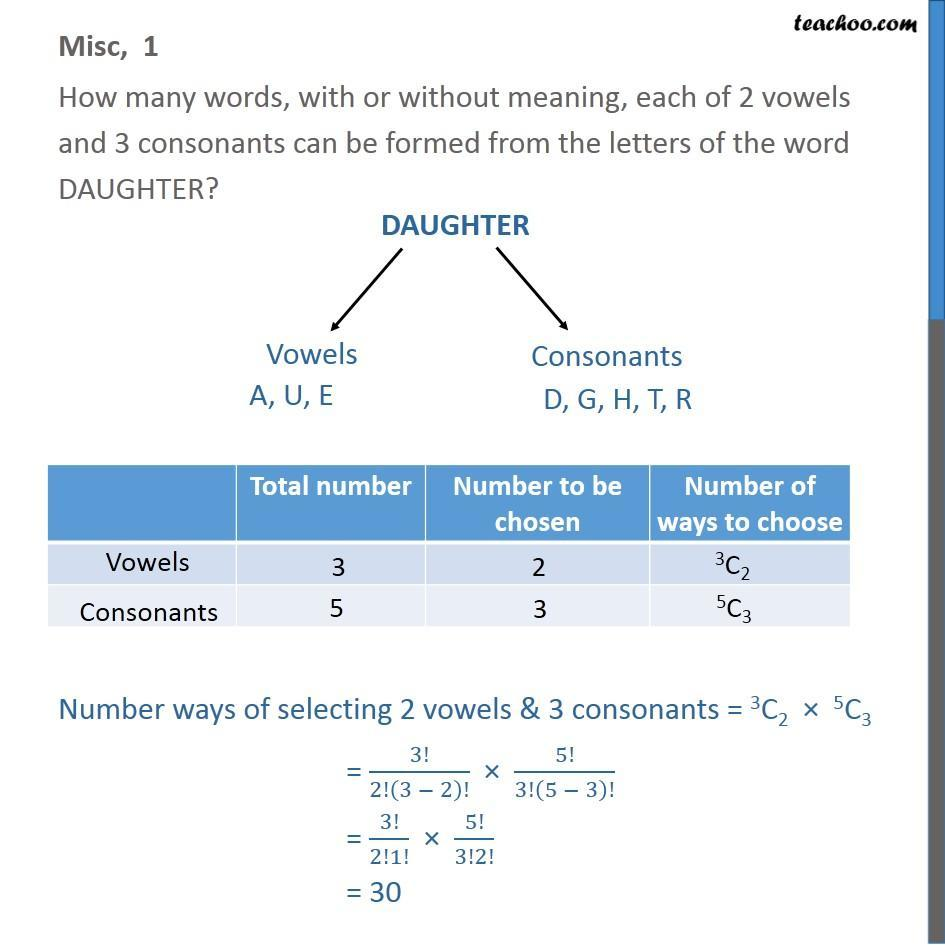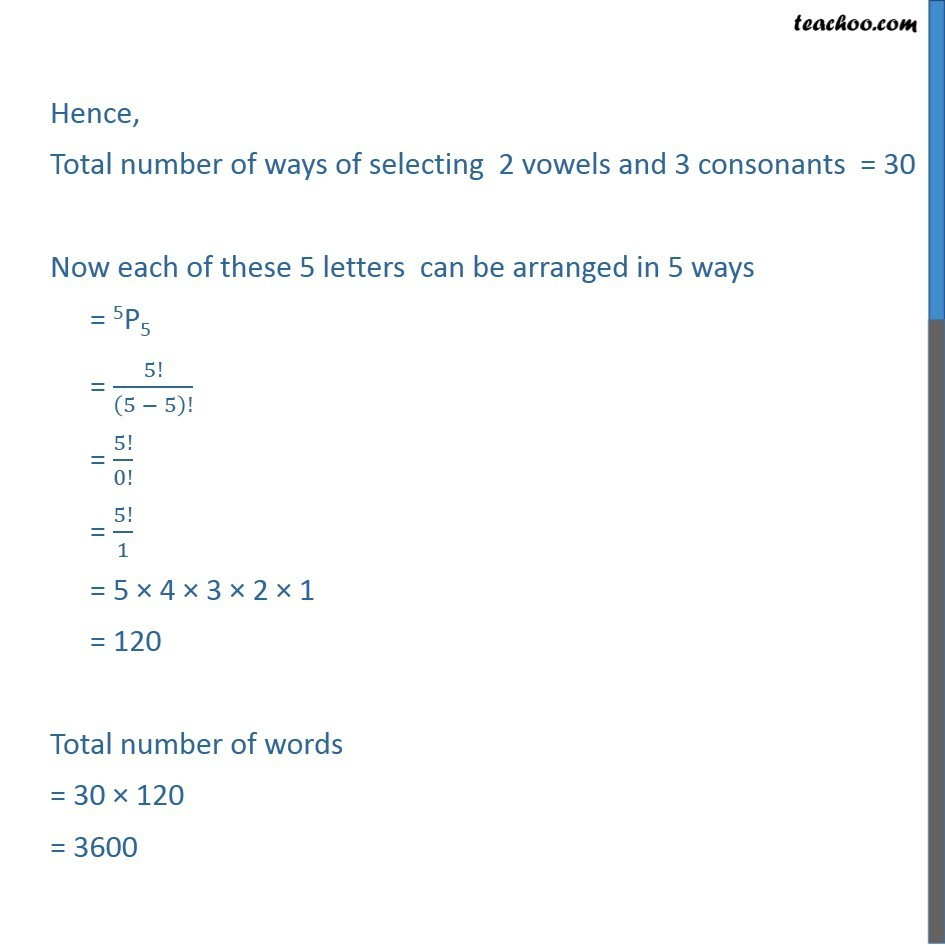1. Chapter 7 Class 11 Permutations and Combinations (Term 2)
2. Concept wise
3. Both Permuation and combination

Transcript

Misc, 1 How many words, with or without meaning, each of 2 vowels and 3 consonants can be formed from the letters of the word DAUGHTER? Hence, Total number of ways of selecting 2 vowels and 3 consonants = 30 Now each of these 5 letters can be arranged in 5 ways = 5P5 = 5!/(5 5)! = 5!/0! = 5!/1 = 5 4 3 2 1 = 120 Total number of words = 30 120 = 3600